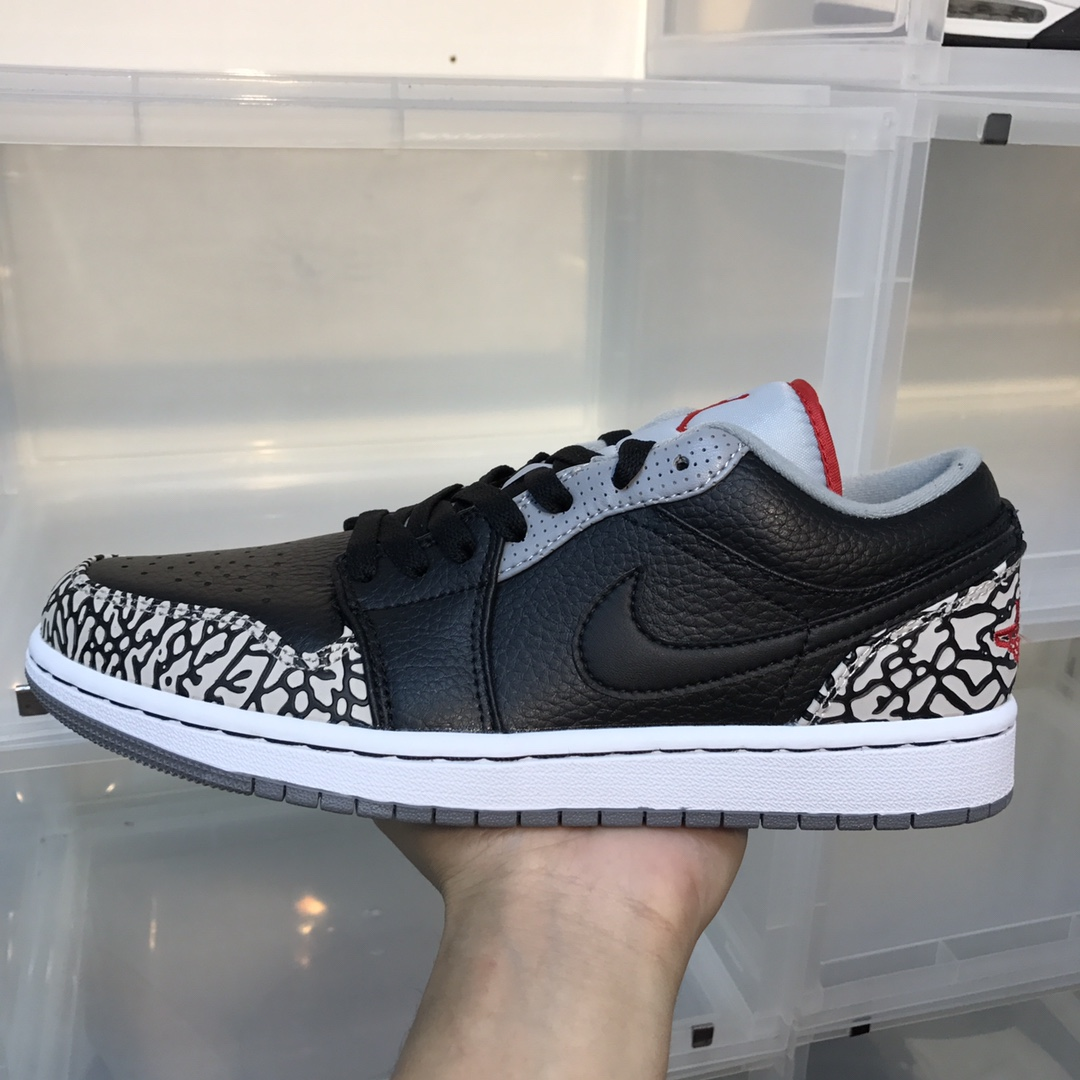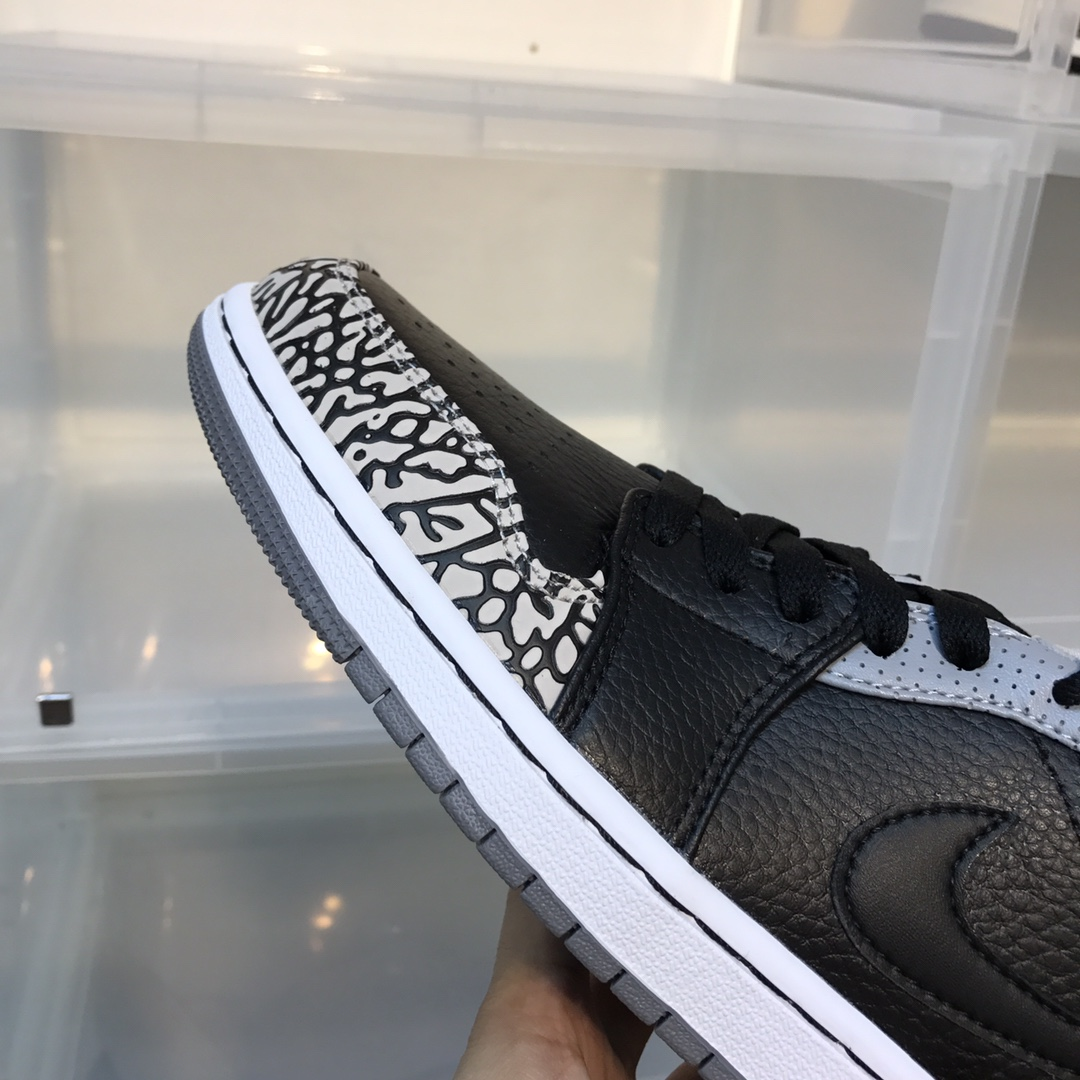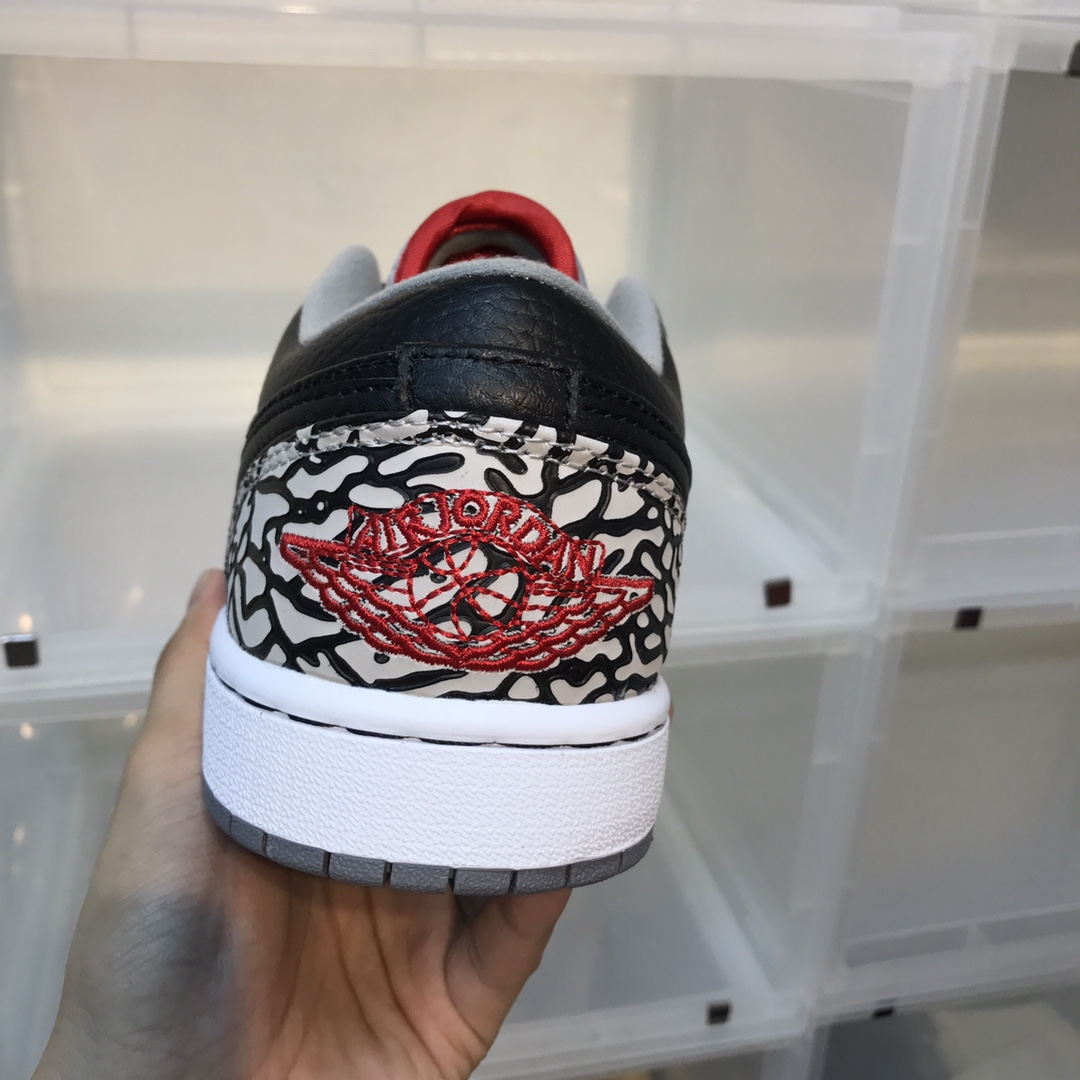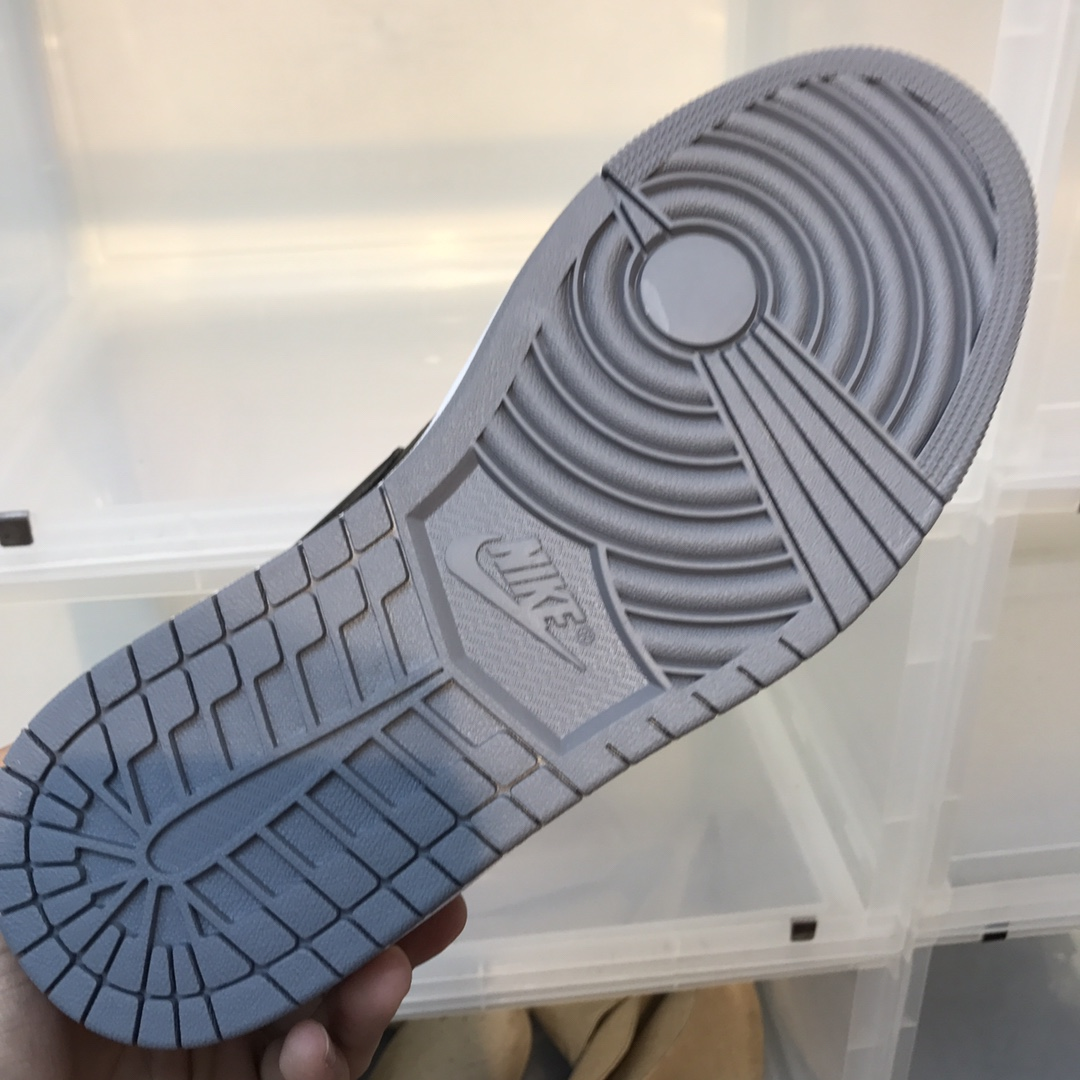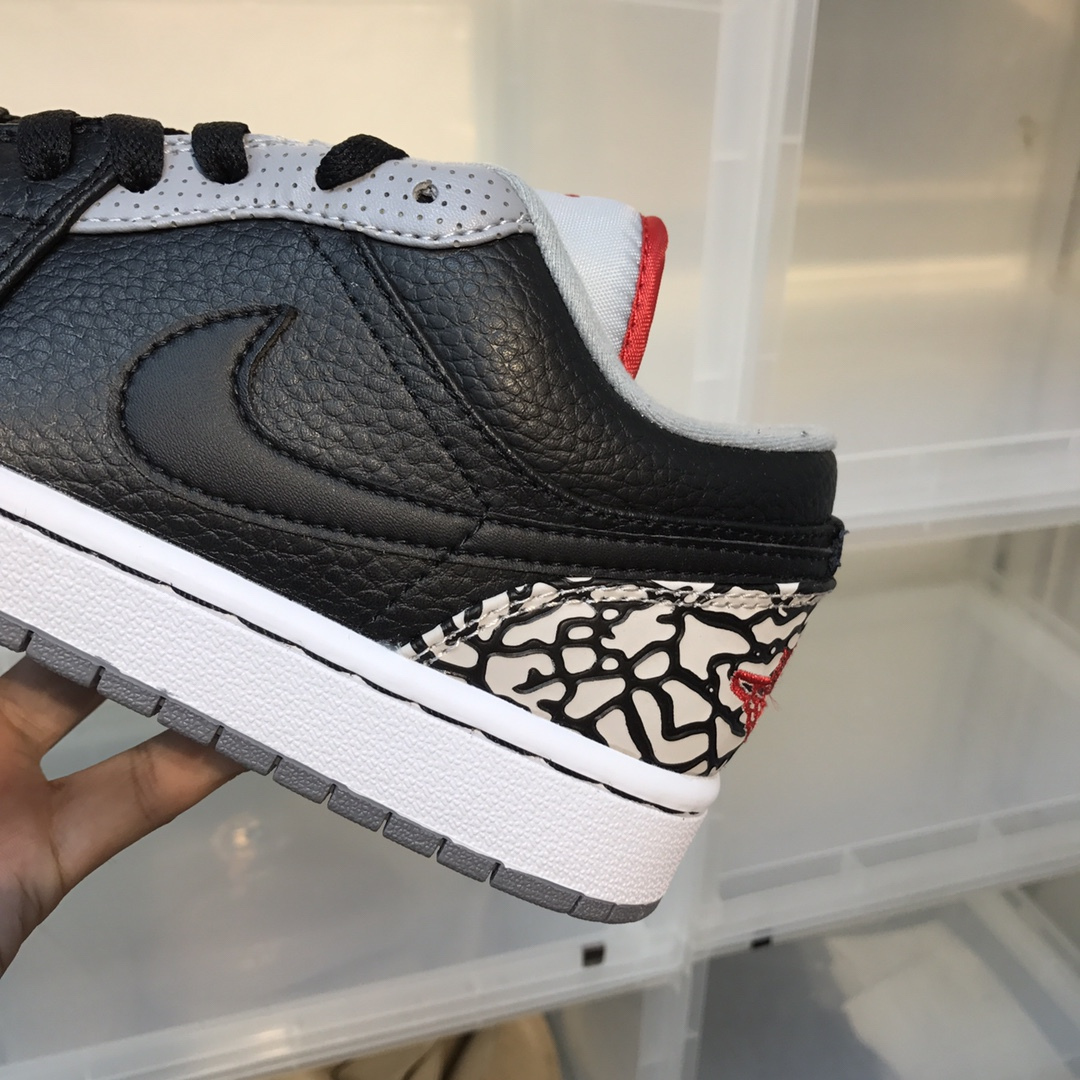Currency : USD

# Air Jordan 1 LowItem NO.: 16830494

USD 160.00 USD 320.00
Size
US4=UK3.5=EUR36=CM23
US4.5=UK4=EUR36.5=CM23.5
US5=UK4.5=EUR37.5=CM23.5
US5.5=UK5=EU38=CM24
US6=UK5.5=EU38.5=CM24
US6.5=UK6=EUR39=CM24.5
US7=UK6=EUR40=CM25
US7.5=UK6.5=EUR40.5=CM25.5
US8=UK7=EUR41=CM26
US8.5=UK7.5=EUR42=CM26.5
US9=UK8=EUR42.5=CM27
US9.5=UK8.5=EUR43=CM27.5
US10=UK9=EUR44=CM28
US10.5=UK9.5=EUR44.5=CM28.5
US11=UK10=EUR45=CM29
US12=UK11=EUR46=CM30
QuantityAir Jordan 1 Low
color:red,size:xxl
Subtotal: USD 0
View My Cart

Copy
•